American Journal of Modern Energy
Volume 2, Issue 6, December 2016, Pages: 58-62

Review Article

An Assessment of Wind Power Density in South East Nigeria, Enugu

A. Ahmed

Department of Mechanical Engineering, Kano University of Science and Technology, Wudil, Nigeria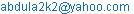A. Ahmed. An Assessment of Wind Power Density in South East Nigeria, Enugu. American Journal of Modern Energy. Vol. 2, No. 6, 2016, pp. 58-62. doi: 10.11648/j.ajme.20160206.15

Received: August 29, 2016; Accepted: December 9, 2016; Published: January 16, 2017

Abstract: The aim of this study is to establish the wind power potential for the wind energy resource in Enugu, south east Nigeria. For this purpose, monthly wind speed data were obtained at 10m height from Nigeria Meteorological station NIMET, Abuja for the period (1990 – 2006). The monthly average values of wind speed, standard deviation, Weibull parameters and wind power were determined. The Weibull and Rayleigh probability density function and the cumulative distribution function respectively were also evaluated. The results show that this region, according to wind power classification is in wind power class II because values of wind power density is greater than 100W/m2. From the results obtained electricity generation from wind power is quite promising for the installation of wind turbines.

Keywords: Power Density, Nigeria, Wind Class, Rayleigh, Weibull

Contents

1. Introduction

Renewable energy sources such as solar thermal, geothermal, biomass, tide and wind power are receiving as effective ways to mitigate energy shortage and reduce environmental pollutions. Wind energy is the fastest developing technologies across the globe due to the advantages of rich resources, wide distribution and environmental friendly .

The rapid growth of wind energy industry led to the production increase and the cost reduction challenges. There are various ways to increase the production and reduce the cost, such as the site selection and site layout design .

In general wind power prediction methods can be classified into two types: physical and statistical. The first ones consider the physical factors such as topography, terrains, local temperature and pressure to estimate the wind power potential. The latter ones predict the wind power potential through statistical model that establish the relationship between the wind power and other variables. Both types of methods have their own virtues: the physical models accurately predict events in the near time horizon. Unlike the wind power prediction method, another wind power assessment method is based on the measured data .

For effective evaluation of wind power available in a particular site, studying the wind statistical characteristics is needed based on this reason, a large number of studies have been published amongst which are: Ahmed et al  presented work on wind energy potential evaluation in northern and southern regions of Nigeria on the basis of the Weibull and Rayleigh models. The work studies 17years measured monthly wind speed and from his results northern region was found to have the highest wind potential as compared to the southern region. Akpinar  presented a work on statistical analysis of wind energy potential on the basis of the Weibull and Rayleigh distribution for Agin-Elazig, Turkey. The work studies 5 years measured wind speed data based on the Weibull and Rayleigh models. The Weibull distribution provides better power density estimation in all twelve months than the Rayleigh models. Emami  worked on the statistical evaluation of wind speed and power density in the Firouzkouh region in Iran. The work studies an hourly average wind data which was observed for one year (2003) at Firouzkouh meteorological station, from his result he found that the region is quite favourable for wind power generation.

The main objective of this present study is to determine the wind energy potential using the Weibull and Rayleigh distribution functions in south east Nigeria, Enugu.

2. Wind Speed Data Collection and Analysis

In the present study, monthly wind speed data for Enugu South East Nigeria covering the period (1990-2006) have been analyzed. The wind speed data were recorded continuously at a height of 10m using a cup generator anemometer and was obtained from Nigeria Metrological Agency (NIMET), Abuja.

Enugu is the largest city located in heart of South Eastern part of Nigeria having latitude 6°26'25N and longitude 7°29'39E. It is characterized by a tropical rain forest with humid climate, mean density temperature of 26.7° and an estimated population of 3.8 million in 2012 with an elevation of 247metres and coal is one of the major natural resource found in the state.

Figure 1 shows wind map for the chosen region. Enugu, it can be observed from the figure that various locations are identified with different colours, this shows that each locations as shown has a range of wind speed speed. This will enable easier investigation of that particular location, having evaluated the site, the researcher can know the type of application to adopt in such locations.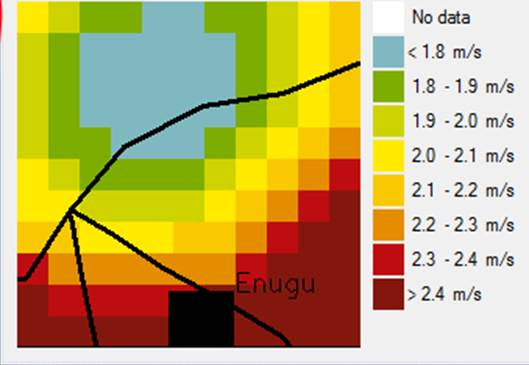Figure 1. Map of various locations of wind speed in Enugu Nigeria.

The continuously recorded wind speed values and the standard deviations can be calculated from the available monthly wind speed data using equations (1) and (2) shown below ;(1)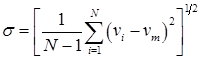(2)

Where vm (m/s) is wind mean speed, Nis the number of months in the period considered, and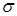(m/s) is the standard deviation.

Weibull and Rayleigh Wind Speed Probability Distribution

The Weibull distribution method is widely accepted for evaluating local wind load and probabilities and is considered as a standard approach. This method has great flexibility and simplicity. However, the main limitation of the Weibull density function is its inability to accurate calculates the probabilities of observing zero or very low wind velocities . Weibull parameter distribution function does not address the differences of wind velocity variation during the course of the day. Nevertheless, this statistical method is found to fit a wide collection of recorded wind data. The Weibull distribution is often used in the field of life data analysis due to its flexibility ; it can mimic the behavior of the other statistical distributions such as the normal and exponential. The probability density function and cumulative function of the Weibull distribution are evaluated using equation (3) and (4) which is given by ;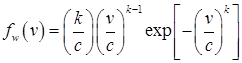(3)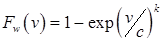(4)

The Weibull parameters (scale and shape factors) can be calculated analytically from the available wind speed data using the relations below (5) given by; ;(5)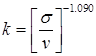(6)

Rayleigh model is a special and simplified case of the Weibull model. It is obtained when the shape factor (k) of the Weibull model is assumed to equal to 2as shown in equation (6). The probability density and the cumulative distribution functions of the Rayleigh model are evaluated from equations (7) and (8) and are given by ;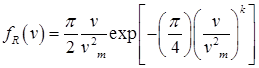(7)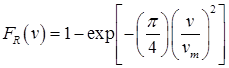(8)

Where fW is Weibull probability density function, FW is the Weibull cumulative distribution function, fR is the Rayleigh probability density function, FR is Rayleigh cumulative distribution function, c is the scale factor (m/s) and k is the shape factor (dimensionless).

3. Analysis of Wind Speed Data

The wind speed probability distribution is in time series usually arranged in the frequency distribution format. The available time series data were translated into frequency distribution format, as shown in Table 1. The wind speed is grouped into classes (bins) as given in the second column of Table 1. The mean wind speeds are calculated for each speed intervals (third column). The fourth column gives the frequency of occurrence of each speed class. The probability density distribution is presented in fifth column. The probability density function for Weibull and Rayleigh are presented in sixth and seventh column, the cumulative distribution function for both Rayleigh and Weibull are present in eighth and ninth column.

Table 1. Arrangement of the measured monthly series data in frequency distribution for Enugu in month of January.

 j Vj vwj fj f(vj) fW(vj) fR(vj) FR(v) FW(V) 1 0 – 0.9 0.45 0 0.000 0.0076714 4.18531E-58 1 0.001421644 2 1 – 1.9 1.45 0 0.000 0.0399527 9.10658E-06 1 0.024133672 3 2 – 2.9 2.45 0 0.000 0.0794243 0.015790068 0.9898 0.083681268 4 3 – 3.9 3.45 0 0.000 0.1156881 0.077337937 0.901 0.181897452 5 4 – 4.9 4.45 2 0.118 0.1401883 0.116958662 0.751 0.311097769 6 5 – 5.9 5.45 8 0.471 0.1477691 0.123934141 0.6042 0.456578053 7 6 – 6.9 6.45 3 0.176 0.1381089 0.115345279 0.484 0.600835582 8 7 – 7.9 7.45 4 0.235 0.1154653 0.102041674 0.391 0.728438255 9 8 – 8.9 8.45 0 0.000 0.0866879 0.088581694 0.3199 0.829723746 10 9 – 9.9 9.45 0 0.000 0.0585195 0.076516649 0.2653 0.902043475 11 10 – 10.9 10.45 0 0.000 0.0355138 0.066188671 0.2228 0.948517316 12 11 – 11.9 11.45 0 0.000 0.019355 0.057503412 0.1894 0.975379276 13 12 – 12.9 12.45 0 0.000 0.009458 0.050237391 0.1627 0.989326467 14 13 – 13.9 13.45 0 0.000 0.0041361 0.044153415 0.1412 0.995820548 15 14 – 14.9 14.45 0 0.000 0.0016153 0.039039471 0.1235 0.998526872

3.1. Wind Power Density

The power of wind can be estimated by using the equation (9) below, given by ;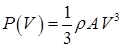(9)

where P is the power in Watts, A is the area perpendicular to the wind speed vector in m2, V is the wind speed in m/s, and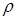is the average density of the air in kg/m3.

Wind power density of a site based on the Weibull and Rayleigh models can be expressed using equation (10) and (11) respectively ;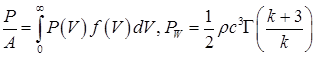(10)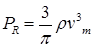(11)

Where PW is Weibull power, PR is Rayleigh power, and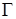is the gamma function.

3.2. Error in Calculating Power Density

The errors in calculating the power densities using the models in comparison to those using the measured probability density distribution and the yearly average error can be obtain using equation 12 and 13 respectively, ;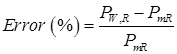(12)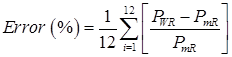(13)

4. Results and Discussion

Table 2 presents the basic information of the wind energy resources for Enugu south east Nigeria which are; monthly average values for the wind speeds, standard deviation and the Weibull parameters. From the table, the values of (c and k) vary from 4.57 - 6.88m/s and 2.43 - 16.59 respectively. The values of mean wind speed vary from 4.42 – 6.47m/s while for standard deviation values vary from 0.35 – 2.63m/s.

Table 2. Average monthly values for wind speed, standard deviation, scale and shape factors.

 Month Jan Feb Mar Apr May Jun Jul Aug Sep Oct Nov Dec vm (m/s) 5.92 5.68 6.14 6.47 5.54 5.34 5.71 5.34 5.14 4.56 4.42 5.34(m/s) 2.63 1.59 1.50 0.76 0.67 0.47 0.45 1.85 0.44 0.35 0.71 1.58 c (m/s) 6.88 6.28 6.72 6.72 5.76 5.42 5.74 5.96 5.20 4.57 4.69 5.92 k 2.43 4.01 4.65 10.26 9.98 14.31 15.81 3.18 14.71 16.59 7.37 3.78

Figure 2 shows the probability density function for Weibull and Rayleigh, it can be seen from the graph that Weibull returns the highest value of 0.1478 as compared to Rayleigh with highest value of 0.1239.Figure 2. Probability density function for Weibull and Rayleigh model.

It can be seen from figure 3 that the mean reference power values are lower as compared to Weibull and Rayleigh power. The Rayleigh model returns higher values in wind power density than the Weibull model in all the months. From the graph the highest power occurred in the month of April for Raleigh model with value of 316.78W/m2.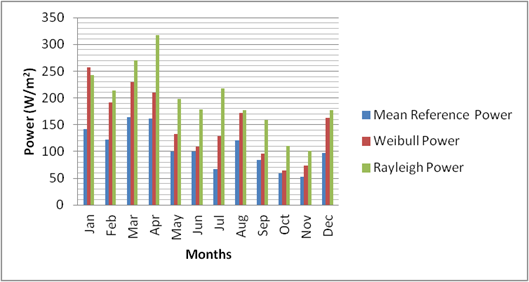Figure 3. Mean reference, Weibull and Rayleigh power (W/m2).

Table 3 presents the monthly percentage error for both Weibull and Rayleigh models, it can be clearly observed that Rayleigh model returns highest percentage in almost all the months except in the month of January were Weibull model have a higher percentage with value 81.3% as compared to Rayleigh model with a corresponding value of 71.8%.

Table 3. Monthly % Error for Weibull and Rayleigh models.

 Month Jan Feb Mar Apr May Jun Jul Aug Sep Oct Nov Dec Weibull(% Error) 81.3 56.9 40.5 30.0 32.9 8.7 91.9 42.3 13.5 9.7 38.2 65.8 Rayleigh(% Error) 71.8 75.4 65.2 95.7 98.5 78.6 98.7 47.0 87.6 86.8 88.8 81.6

5. Conclusion

In this research work, monthly measured series wind speed data for Enugu South East Nigeria have been analyzed. The probability density distributions have been derived from the monthly series data and the distributional parameters were identified. Two probability density functions have been fitted to the measured probability distributions on a monthly basis. The wind energy potentials of the locations have been studied based on the Weibull and Rayleigh models. From the wind speed data analysis and calculations, we can conclude that;

(1)  In Enugu south east Nigeria, the highest average monthly wind speed was determined to be 6.47m/s in month of April while lowest wind speed occurred in month of November with value of 4.42m/s.

(2)  The average value for Weibull parameters (k and c) in Enugu varies between 2.43 – 16.59 and 4.57 – 6.88m/s respectively.

(3)  The location is suitable for electricity generation since the annual average power density for both Weibull and Rayleigh were obtained to be 152.35W/m2 and 197.02 W/m2 respectively, this indicates that Enugu correspond to wind power class II, since the average wind power density value is greater than 100W/m2. In this location wind energy conversion system (WECS) can be installed to supply a reasonable amount of energy using a number of wind turbines.

(4)  The Weibull distribution provides better power estimation in almost all 12 months than the Rayleigh model because the Weibull model returns lower error in power estimation.

(5)  In calculating the error in power estimation for both models, the Weibull model returns smaller errors in power density estimation than the Rayleigh model.

Finally, Enugu south east Nigeria have wind energy potential that is quite promising for installation of wind turbines.

References

1. Ahmed A, Bello AA, Habou D. An evaluation of wind energy potential in the northern and southern regions of Nigeria on the basis of Weibull and Rayleigh models. American Journal of Energy Engineering 2013; 1: 37–42.
2. Ahmed A, El-Suleiman A, Nasir A. An assessment of wind energy resource in north central Nigeria, Plateau. Science Journal of Energy Engineering 2013; 1: 13-17.
3. Akpinar EK, Akpinar S. Statistical analysis of wind energy potential on the basis of the Weibulland Rayleigh distribution for Agin-Elazig Turkey. Power and Energy 2004; 218: 557–565.
4. Alsaad, MA. Wind energy potential in selected areas in Jordan. Energy Conversion and Managment 2013; 65: 704–708.
5. Chen D, Song MX, Zhang X. A statistical method to merge wind cases for wind power assess-ment of wind farm. Journal of Wind Engineering and Industrial Aerodynamics 2013; 119: 69–77.
6. Chen D, Zhang W. Exploitation and research on wind energy. Energy Conversion Technology2007; 4: 339–343.
7. Emami N, Behbahani-Nia A. The statistical evaluation of wind speed and power density in theFirouzkouh region in Iran. Energy Sources 2012; 34: 1076–1083.
8. Jie W, Jianzhou W, Dezhong C. Wind energy potential assessment for the site of Inner Mongoliain China. Renewable and Sustainable Energy Reviews 2013; 21: 215–228.
9. Landberg L. Short-term prediction of power production from wind farms. Journal of Wind Engineering and Industrial Aerodynamics 1999; 80: 207–220.
10. Stathopoulos C, Kaperoni A, Galanis G, Kallos G. Wind power prediction based on numericaland statistical models. Journal of Wind Engineering and Industrial Aerodynamics 2013, 112: 25-38.
11. Wiser R, Bolinger M. Annual report on U.S. Wind power installation, cost and performance trend2006, NREL, US Department of Energy, Golden, Co: Available at:(http://www.nrel.gov/wind/pdfs/41435.pdf).

 Contents 1. 2. 3. 3.1. 3.2. 4. 5.
Article ToolsAbstractPDF(688K)